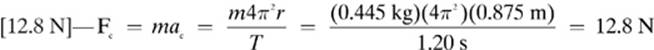﻿ ﻿Answer Key - Rotational and Circular Motion - Homework Helpers: Physics

## 4 Rotational and Circular Motion

The actual answers will be shown in brackets, followed by an explanation. If you don”t understand an explanation that is given in this section, you may want to go back and review the lesson that the question came from.

Lesson 4–1 Review

1.2.3.Lesson 4–2 Review

1. [torque]—The formula for torque is: τ = dF sin θ

2.3. [9.28 N · m]—τ = Fd sin θ = (18.5 N)(0.875 m)(sin 35.0°) = 9.28 N · m

4. [greater torque]—When you have a longer wrench you can exert a force at a greater distance from the axis of rotation, resulting in greater torque. Many people keep a pipe in their car which they can attach to the end of their wrench to increase the length of the lever arm.

Lesson 4–3 Review

1. [Tangential speed]

2.3. [4.47 m/s]—v = = (0.994 m)(4.50 rad/s) = 4.47 m/s

Lesson 4–4 Review

1. [Centripetal force]—The formula for centripetal force is Fc = mac.

2. [1,530 N]—Fc = mac = (65.0 kg)(23.5 m/s2) = 1,530 N

3.Lesson 4–5 Review

1. [Field force]—Example of field forces are gravity, electrostatics, and magnetism.

2. [50 N]—Newton”s law of universal gravitation tells us that the force of gravitational attraction between two objects due to gravity is inversely proportional to the distance between them. If you double the distance between the objects, the measurement of the weight will be divided by3. [783 N]—Do you remember the difference between mass and weight? The weight of an object changes due to location, but the mass doesn”t. Let”s begin by finding the mass of the man, which will be the same at any altitude.Next, let”s add the altitude of the aircraft to the radius of Earth to find the total distance between the man and the center of Earth.

6.37 × 106 m + 1.00 × 104 m = 6.38 × 106 m

Now we can use Newton”s law of universal gravitation to calculate the weight of the man at this new distance.Lesson 4–6 Review

1. [gravity]—It is the gravitational attraction between the Earth and the sun that keeps the Earth in orbit.

2. [perihelion]—Think of “away” for “aphelion.”

Chapter 4 Examination

1. [c. period]—If you get period mixed up with frequency, just think of “period of time.”

2. [i. circular motion]

3. [a. radian]—One radian also equals the angle subtended at the center of a circle by an arc length equal to the length of the radius of the circle.

4. [g. hertz]—Frequency is measured in hertz.

5. [b. torque]—The torque is the product of the component of the force that causes rotation, and the length measured between the location of the force and the axis of rotation.

6.7.8.9.10. [d. 2.75 m/s]—v = = (0.350 m)(7.85 rad/s) = 2.75 m/s

11.12.13.decreases with the square of the distance. 32 = 9, so the force is divided by 9.

14. [b. 1.2 kg]—Remember, G = 6.67 × 10–11 N · m2/kg2

Isolating m2 in our equation for universal gravitation, we get15. [20.4 m/s2]—ac = 2 = (1.35 m)(3.89 rad/s)2 = 20.4 m/s2

16. [1.5 N]—The key to this problem is to make sure you don”t confuse position with distance. We need to find out how far the forces are going to be exerted from the pivot point.Note: You may have been surprised that I didn”t convert centimeters to meters before solving the problem. However, as you can see from the solution, these units will cancel out, making the conversions unnecessary.

17.18.19. [297 N]—You need to calculate the value of the acceleration due to gravity (gmars) on Mars, and multiply this value by the man”s mass.

20.﻿